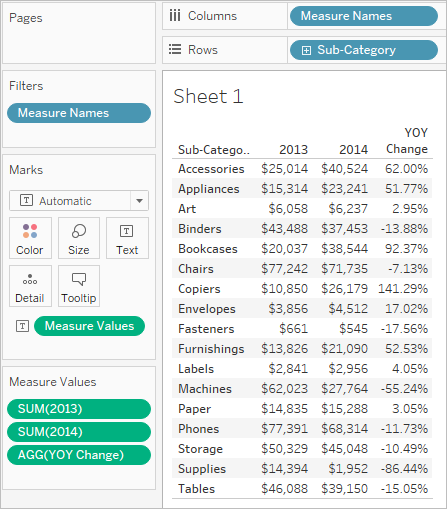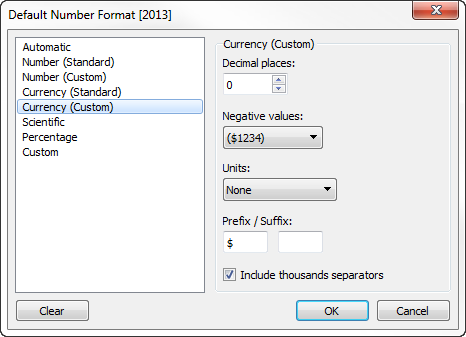# Add a Calculated Column to a View

Sometimes, columns in your text table do not allow you to display the results of certain calculations as you might expect. In cases like this, you can create a calculated column that uses a single formula that automatically adjusts the value for each row in the table. For example, suppose you want to create a view that displays the sales for each year in several columns and the year-over-year (YOY) percentage change in the final column. There are different ways to get the result you want, but a fairly straightforward approach is to create create custom calculations use them in the view.

## Objective

To create a view like the following, that shows sales results for two years in the first two columns, and then the year-over-year change, as a percentage, in the third column.## How to Build It

The scenario uses the Sample - Superstore data source provided with Tableau Desktop to show how to build the view shown above.

### Create the necessary calculated fields

1. Connect to the Sample - Superstore data source.

2. Choose Analysis > Create Calculated Field to open the calculation editor. Name the calculation 2013 and type or paste the following in the formula area:

`IF YEAR([Order Date]) = 2013 THEN [Sales] ELSE 0 END`

3. Create a second calculated field and name it 2014; the formula is the same except with 2014 instead of 2013:

`IF YEAR([Order Date]) = 2014 THEN [Sales] ELSE 0 END`

4. Create a third calculated field, YOY Pct. Change, to calculate the change from 2013 to 2014:

`(SUM() - SUM()) / SUM()`

### Format the calculated fields

1. Click 2013 in the Measures area of the Data pane and choose Default Properties > Number Format.

2. In the Default Number Format dialog box, set the format to Currency (Custom) and the Decimal places to 0.3. Format the 2014 measure the exact same way.

4. Format the YOY Pct. Change field as Percentage, with 2 decimal places.

### Build the view

1. Drag 2013 to Text on the Marks card.

2. Double-click 2014 and then YOY Pct. Change.

3. Drag Measure Names from Rows to Columns.

4. Drag Sub-Category to Rows.

Your view should now look like the one at the beginning of this topic.